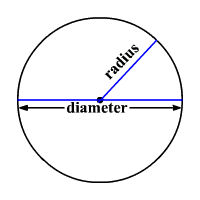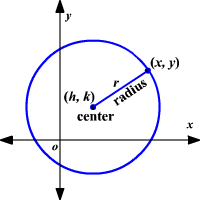# Equation of a Circle

A circle is the set of all points in a plane at a given distance (called the radius ) from a given point (called the center.)A line segment connecting two points on the circle and going through the center is called a diameter of the circle.

Assume that $\left(x,y\right)$ are the coordinates of a point on the circle shown. The center is at $\left(h,k\right)$ , and the radius is $r$ .Use the Distance Formula to find the equation of the circle.

$\sqrt{{\left({x}_{2}-{x}_{1}\right)}^{2}+{\left({y}_{2}-{y}_{1}\right)}^{2}}=d$

Substitute $\left({x}_{1},{y}_{1}\right)=\left(h,k\right),\left({x}_{2},{y}_{2}\right)=\left(x,y\right)$ and $d=r$ .

$\sqrt{{\left(x-h\right)}^{2}+{\left(y-k\right)}^{2}}=r$

Square each side.

${\left(x-h\right)}^{2}+{\left(y-k\right)}^{2}={r}^{2}$

The equation of a circle with center $\left(h,k\right)$ and radius $r$ units is ${\left(x-h\right)}^{2}+{\left(y-k\right)}^{2}={r}^{2}$ .